O 1 O O 1 O O 1 O 1 1 O 1 1 O 1 O 1 1 O O O O 1 O 1 1 O O 1 1 1 O 1 1 O 1 O O 1 O 1 1 O 1 1 1 O O 1 1 O O O O 1 O 1 1 1 O 1 O O O 1 1 O 1 O O 1 O 1 1 O 1 1 1 1 O 1 1 O 1 1 1 O O O 1 O O O O O O 1 1 O 1 O O 1 O 1 1 1 O O 1 1 O O 1 O O O O O O 1 1 O 1 1 O 1 O 1 1 O 1 1 1 1 O 1 1 1 O O 1 O O 1 1 O O 1 O 1 O O 1 O O O O O O 1 1 O 1 O O 1 O 1 1 O 1 1 O 1 O 1 1 1 O O O O O 1 1 O 1 1 1 1 O 1 1 1 O O 1 O O 1 1 1 O 1 O O O 1 1 O O O O 1 O 1 1 O 1 1 1 O O 1 1 1 O 1 O O O O 1 O O O O O O 1 1 1 O 1 O O O 1 1 O 1 O O O O 1 1 O O O O 1 O 1 1 O 1 1 1 O O O 1 O O O O O O 1 1 O 1 O 1 1 O 1 1 O 1 1 1 O O 1 1 O 1 1 1 1 O 1 1 1 O 1 1 1 O 1 1 O 1 1 O O O 1 1 O O 1 O 1 O 1 1 O O 1 O O O 1 1 O O 1 1 1 O 1 1 O O 1 O 1 O O 1 O 1 1 1 O O O 1 O O O O O O 1 O O O 1 1 O O 1 1 O 1 1 1 1 O 1 1 1 O O 1 O O O 1 O O O O O O 1 1 O 1 O 1 1 O 1 1 O 1 1 1 O O 1 1 O 1 1 1 1 O 1 1 1 O 1 1 1 O 1 1 O 1 1 O O O 1 1 O O 1 O 1 O 1 1 O O 1 O O O 1 1 O O 1 1 1 O 1 1 O O 1 O 1 O O 1 O O O O O O 1 1 O 1 O O 1 O 1 1 1 O O 1 1 O O 1 O O O O O O 1 1 O 1 1 O O O 1 1 O 1 O O 1 O 1 1 O 1 1 O 1 O 1 1 O 1 O O 1 O 1 1 1 O 1 O O O 1 1 O O 1 O 1 O 1 1 O O 1 O O O O 1 O O O O O O 1 1 1 O 1 O O O 1 1 O 1 1 1 1 O O 1 O O O O O O 1 1 O O O O 1 O 1 1 O 1 1 O O O 1 1 O 1 1 O O O O 1 O O O O O O 1 1 1 O 1 1 1 O 1 1 O O 1 O 1 O O 1 O O O O O O 1 1 O 1 1 1 O O 1 1 O 1 1 1 1 O 1 1 1 O 1 1 1 O O 1 O O O O O O 1 1 O 1 O 1 1 O 1 1 O 1 1 1 O O 1 1 O 1 1 1 1 O 1 1 1 O 1 1 1 O O 1 O O O O O O 1 1 O O O O 1 O 1 1 O 1 1 1 O O 1 1 O O 1 O O O O 1 O O O O O O 1 1 1 O 1 O 1 O 1 1 O 1 1 1 O O 1 1 O O 1 O O O 1 1 O O 1 O 1 O 1 1 1 O O 1 O O 1 1 1 O O 1 1 O 1 1 1 O 1 O O O 1 1 O O O O 1 O 1 1 O 1 1 1 O O 1 1 O O 1 O O O O 1 O 1 1 O O O O 1 O O O O O O 1 1 1 O 1 1 1 O 1 1 O 1 O O O O 1 1 O 1 O O 1 O 1 1 O 1 1 O O O 1 1 O O 1 O 1 O O 1 O O O O O O 1 1 O 1 O O 1 O 1 1 O 1 1 O 1 O 1 1 O O O O 1 O 1 1 O O 1 1 1 O 1 1 O 1 O O 1 O 1 1 O 1 1 1 O O 1 1 O O O O 1 O 1 1 1 O 1 O O O 1 1 O 1 O O 1 O 1 1 O 1 1 1 1 O 1 1 O 1 1 1 O O O 1 O O O O O O 1 1 O O 1 O 1 O 1 1 O 1 1 O 1 O 1 1 O O O 1 O O 1 1 1 O O 1 O O 1 1 O O O O 1 O 1 1 O O O 1 1 O 1 1 O O 1 O 1 O 1 1 1 O O 1 1 O O 1 O O O O O O 1 1 1 O 1 O O O 1 1 O 1 O O O O 1 1 O O 1 O 1 O O 1 O O O O O O 1 1 O O 1 O 1 O 1 1 O 1 1 1 O O 1 1 1 O 1 O O O 1 1 O 1 O O 1 O 1 1 1 O O 1 O O 1 1 O O 1 O 1 O O 1 O O O O O O 1 1 1 O 1 1 1 O 1 1 O 1 1 1 1 O 1 1 1 O O 1 O O 1 1 O 1 1 O O O 1 1 O O 1 O O O O 1 O 1 1 O O O O 1 O O O O O O 1 1 O O O O 1 O 1 1 O 1 1 1 O O 1 1 O O 1 O O O O 1 O O O O O O 1 1 O O O O 1 O 1 1 O 1 1 O O O 1 1 O 1 1 O O O O 1 O O O O O O 1 1 1 O 1 O O O 1 1 O 1 O O O O 1 1 O O 1 O 1 O 1 1 1 O O 1 O O 1 1 O O 1 O 1 O O 1 O O O O O O 1 1 O O 1 O 1 O 1 1 1 O 1 1 O O 1 1 O O 1 O 1 O 1 1 1 O O 1 O O O 1 O O O O O O 1 1 1 O 1 1 1 O 1 1 O 1 O O 1 O 1 1 O 1 1 O O O 1 1 O 1 1 O O O O 1 O O O O O O 1 1 O O O 1 O O 1 1 O O 1 O 1 O O 1 O O O O O O 1 1 1 O 1 O O O 1 1 O 1 1 1 1 O O 1 O O O O O O 1 1 O 1 O 1 1 O 1 1 O 1 1 1 O O 1 1 O 1 1 1 1 O 1 1 1 O 1 1 1 O O 1 O O O O O O 1 1 O O O O 1 O 1 1 O 1 1 1 O O 1 1 O O 1 O O O O 1 O O O O O O 1 1 1 O 1 O 1 O 1 1 O 1 1 1 O O 1 1 O O 1 O O O 1 1 O O 1 O 1 O 1 1 1 O O 1 O O 1 1 1 O O 1 1 O 1 1 1 O 1 O O O 1 1 O O O O 1 O 1 1 O 1 1 1 O O 1 1 O O 1 O O
Clock Widget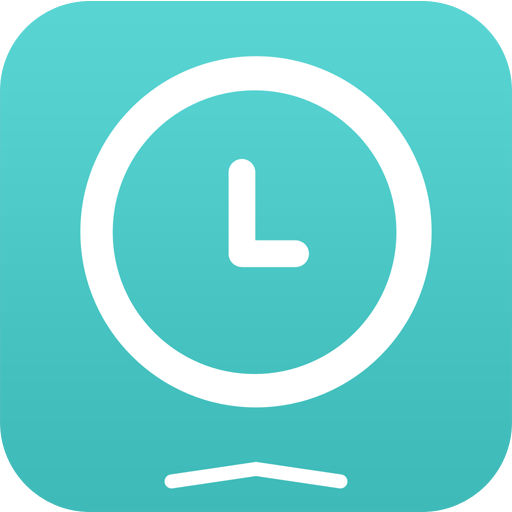# Presentation

Thanks to this application (and the other Widget apps from PacoLabs), you can now customize the Today view of your device, by adding custom widgets for some specific purposes.

Each Clock widget allows to view 1 to 6 different clocks on the same row.
Each clock can display the current time or the one of a specific timezone.
The time can be displayed as an analog or a digital clock, with or without the seconds.
A text can be associated (to display the town name and time offset).
Various parameters can be changed (like the font name and the day or night colors).

The application is really easy to use and is adapted to the fresh look of your device.
Dark mode of iOS13 is supported.
You can add as many widgets as you want and they will be displayed in up to 3 different lists. Those same lists will be available in the Today view and in the application (although it is possible, if you want, to hide some widgets in the Today view).
To avoid overloading the Today view, when too many widgets are displayed, an intuitive control will allow you to navigate from one widget to another.
Even if the main purpose of the app is to view the widgets in the Today view, it can also be very handy to view them directly in the application (a long press on a widget in the Today view will open it directly in the main application).

# iPhone Screenshots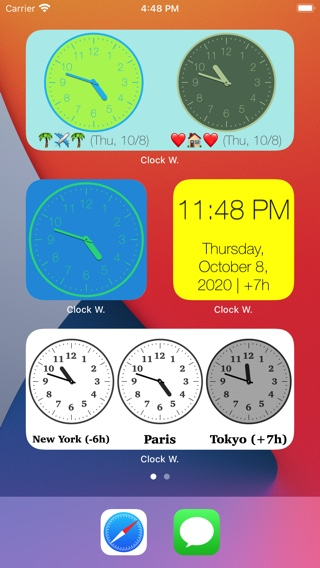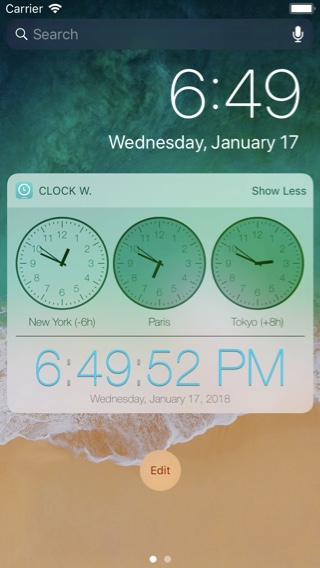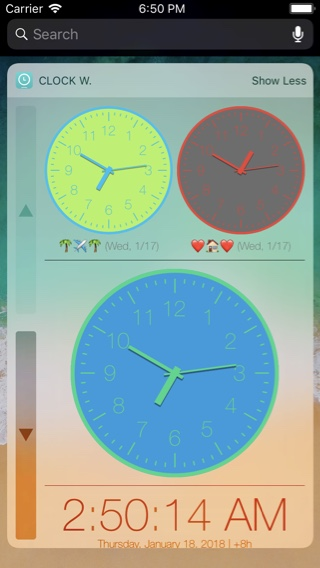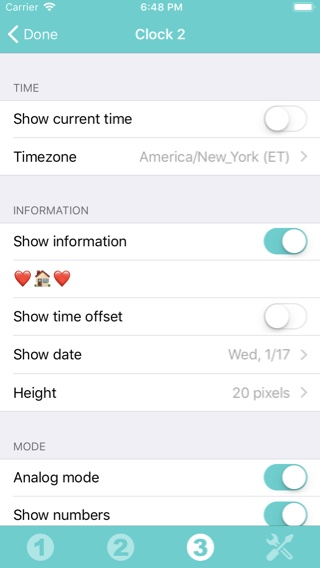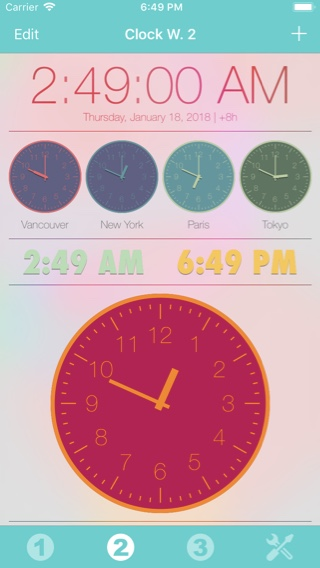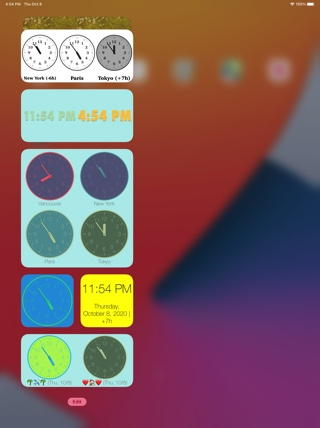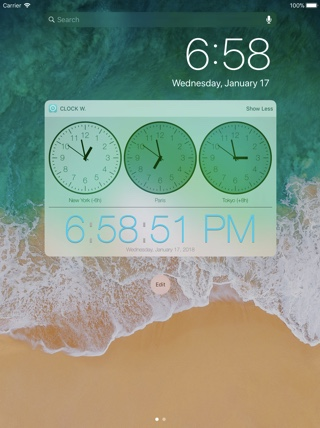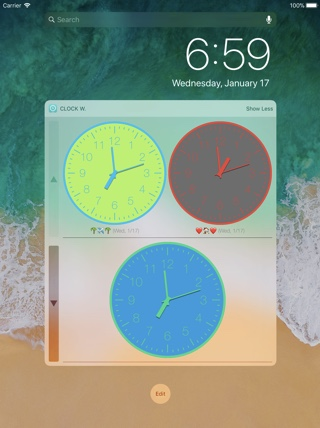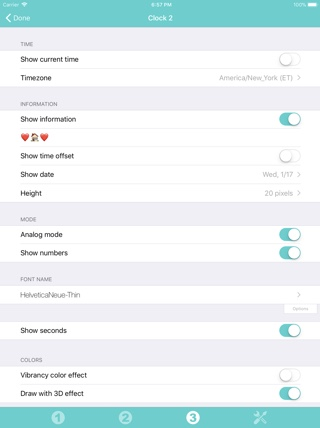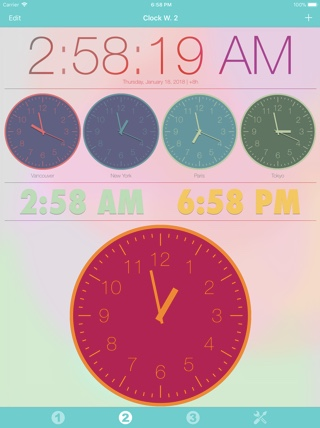# History

## v4.2.2

• Fixed an incorrect layout of the icons in the bottom bar in the app on some phones.
• Fixed a problem preventing the title date of a clock information to be displayed in some specific cases.

# Availability

Clock Widget can be purchased on the App Store